# ISEE Middle Level Math : Operations

## Example Questions

← Previous 1 3 4 5 6 7 8 9 20 21

### Example Question #31 : Algebra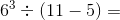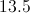Explanation:

First find the exponent value: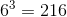Then find the value of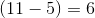Finally, solve the entire expression with the known values: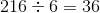### Example Question #642 : Concepts

Simplify: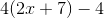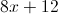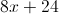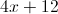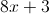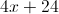Explanation: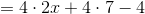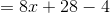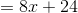### Example Question #2 : Multiplying And Dividing Polynomials

Multiply: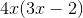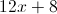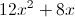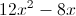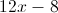Explanation:

Use the distributive property: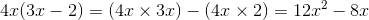### Example Question #643 : Concepts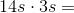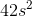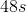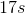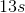Explanation:

Multiply the numbers and multiply the variables: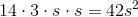Answer:### Example Question #644 : Concepts

Simplify: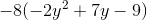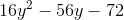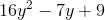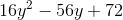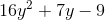Explanation: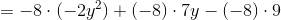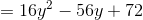### Example Question #645 : Concepts

Multiply: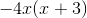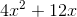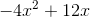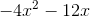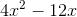Explanation: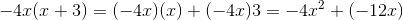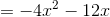### Example Question #646 : Concepts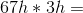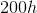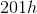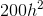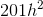Explanation:

Multiply the whole numbers and add an exponent to the variable totaling the number of exponents in the equation: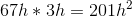Answer:### Example Question #647 : Concepts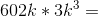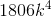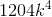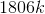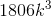Explanation:

Multiply the whole numbers and add an exponent to the variable totaling the number of exponents in the equation: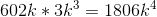Answer:### Example Question #1 : Operations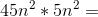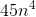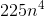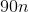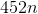Explanation:

Multiply the whole numbers and add an exponent to the variable totaling the number of exponents in the equation: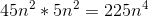Answer:### Example Question #2 : Operations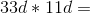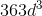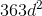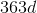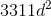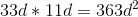Answer: